# Balloon Calculus!

## Standard integrals, derivatives and methods

 Garden City Maths - Online tuition!Find me on Zoom - just open a meeting and invite through email. Or just email, and I'll help set up. email: tom@ballooncalculus.org. Newtonian Mechanics - interactive!
##### Integral/differand

 sec2 x tan x

 -cosec x cot x sec x tan x - cosec2 x cosec x sec x cot xarctan xarcsin x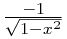arccos x

 tan x ln | sec x |

 cosec x ln | tan (x/2) | sec x ln | sec x + tan x | cot x ln | sin x |

 Differentiate a product Differentiate a composite function (chain rule) Differentiate a quotient Differentiate an inverse trig function Integration by parts Integration by substitution / change of variable Integration by internal substitution Separation of variables Integrating factor Exact equations Reduction of Order Fundamental theorem of calculus Double and Triple Integrals

Given these pairs (either differentiating down or, adding a constant of integration, integrating up)...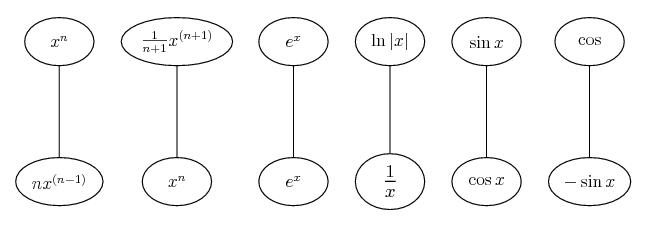... along with the chain rule...... and the product rule...... we illustrate some common results below...

Derivative of tan x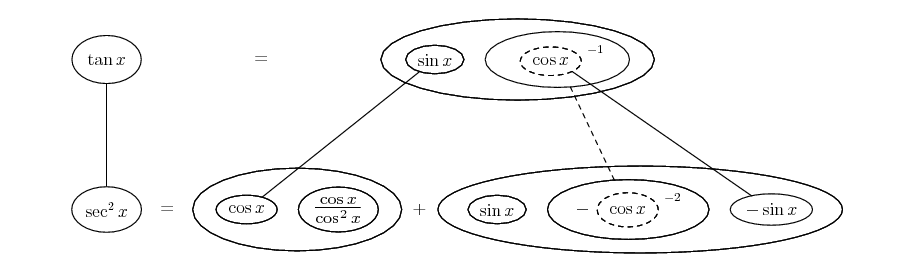Usually, you'll integrate sec2 x by remembering that it's the derivative of tan x. If you want to forego that short-cut and integrate strategically, you can use the Weierstrass sub - see for example the integrate cosec section below.

Derivative of cosec xDerivative of sec x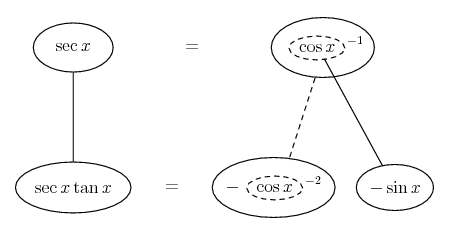Derivative of cot xDerivative of arctan x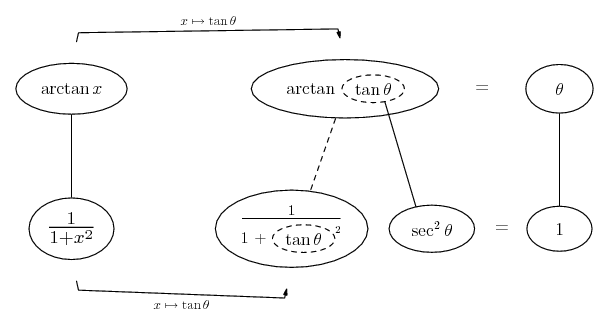Derivative of arcsin xDerivative of arccos xIntegral of tan x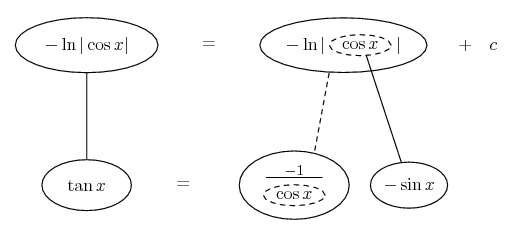Alternatively...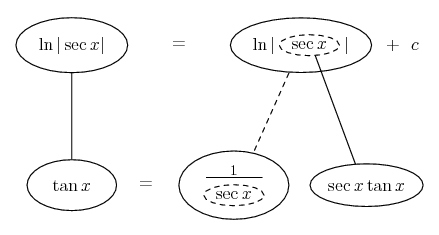Integral of cosec xAlternatively, using the Weierstrass substitution, which is more round about but also a more widely applicable method...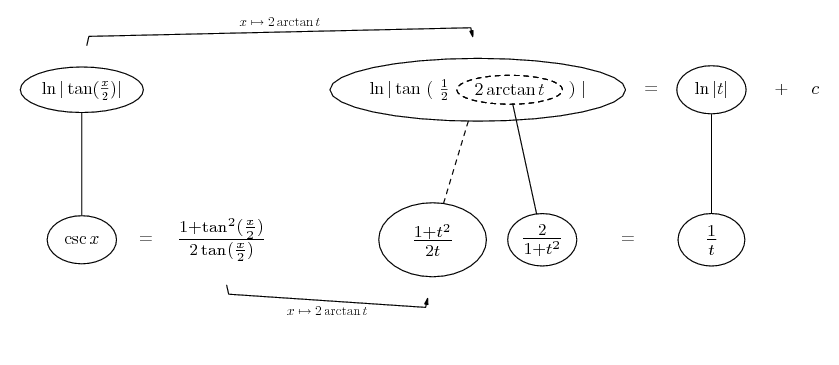Integral of sec x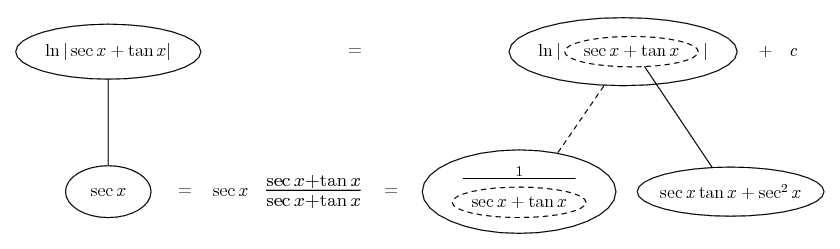Alternatively, using the Weierstrass substitution, which is more round about but also a more widely applicable method...

Integral of cot x##### Differentiate a product... is the product rule. The straight lines differentiate downwards (integrate up) with respect to x (or whatever the main, explicit variable). And then, because of the product rule, the whole of the bottom row is the derivative (with respect to x) of the whole of the top row. Choosing legs crossed or uncrossed is unimportant when differentiating but crucial for integration by parts (see below).

So differentiation is a downwards journey, and if you want to expand the picture with equals signs (often a good idea) then it's probably also clockwise...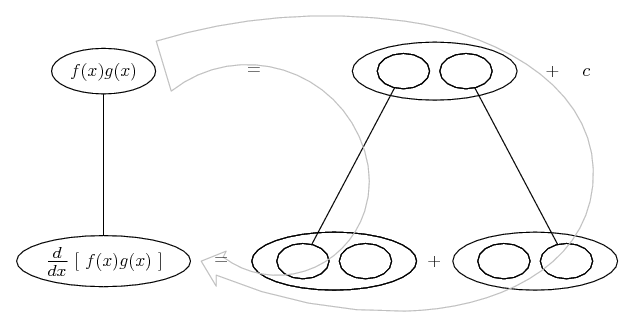Examples of differentiating a product (3x2 - 3) (x2 - 2) / (x2 + 2) x e-kx relativisitic momentum x arctany d2/dt2 et cos t m ln m + em tan m (1 - e-x2) (1 - e-y2) 2x/(y2 + 2) ∂/∂x, ∂/∂y... ex siny f(x - y) x2 e-kx (5x3 + 1)8 (4x5 + 3)7 -8x-x2 tanx ln|2x + 5| t (1 - t2) x2 e-3e x2 (x - 2)2 3x e2x cos4(3t) sin(3t) 2x  ln |x2 + 5| x(1 - x)(3/5)

Related rates production level and price total & average income price, sales and revenue

##### Differentiate a composite function... is the chain rule. Straight continuous lines differentiate downwards (integrate up) with respect to x, and the straight dashed line similarly but with respect to the dashed balloon expression (which is the inner function of the composite expression which is subject to the chain rule).

As with the product rule, differentiation is generally a clockwise journey...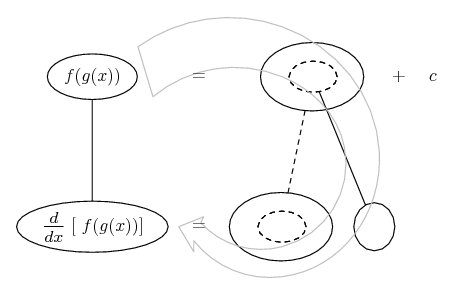Examples of differentiating a composite function ln(tanx) differentiate integral of composite directional derivatives sin3(cos2(3ex2 )) ∂/∂x √ (x2 + y2) f(4x3) 1 / (1 + 12.6e-0.73x) Lorentz factor 3(2 - 8x)4 wave equation 3ex2 sin(e3x) sin2(sin(sinx)) tan2(x3) 5x3 - 3/(2 - 7x) + 3x5 - 15 sec3(π /2 - x) ekx δ / δx exy √(x + √(x + √(x))) arcsin3(2x) [ sin( 1 + {cos( 1 + [tan(1+x)]4) }3) ]2 [(4x + 3) / (5x - 2)]7 esec x (6x4 - 6x -3 - 2x + 5) -2 - ln | cos x | ln | 8x3 - 7x + 2.78 |

Related rates snowball melting resisters in parallel shadow against a wall revenue given rate for cost ladder sliding down wall rate y 12 times rate x tracking balloon rise shadow length at noon camera tracking ferris wheel highway patrol plane area of inkstain track climbing aircraft aeroplanes converging piston, crank, connecting rod outer rate 12 times inner camera tracking train flow through conical tank rope lifting pipe from far end rotating light-house beam coffee filling in truncated cone rotating spot-light ladder towards wall inflating balloon conical sand pile

Double composite
partial derivative proof d/dx 4/3 x3/4 - x Φ(u,v) = f(uv cos v, uv sin v z(u,v) = f(u2 - v2, 2uv) x = u(s,t), y = v(s,t) d/dx (x2)ex d/dt (5 - 3t)4t d/dx (tan x)x d/dx x (1/x) d/dx f(x) g(x) d/dx x sinx d/dx 10x ln x ∂/∂x (x2 - y2) / (4xy3)

Logarithmic differentiation d/dx cosx lnx / x2 d/dx 4/3 x3/4 - x y = 5x2x tan(6x) d/dx x7x

Implicit differentiation x2 + wx + w2 = 1 x2y3 + 2y = 6 x2y + 3x - 2y = 6 y + ey = x - e2x2 y + x ln y = cos(xy2) 2x/(y2 + 2) y + 2x siny = ex d/dt √(x2 + y2) x2y + xy2 = 6 4x3 + 2xy - y3 = 1/2 x ey = y - 1 ex2 + y6 = 2x cos y x2 + y2 = 25 x3y / (1 - xy) 2x2y = sin(2x) x2y = x + y2 sin3x cos2y = 6 3x2 + 4xy3 = 9 6x + 10y dy/dx - 6 + 20 dy/dx x2 - xy + ¾ x2 = 7

Internal substitution differentiate an inverse

##### Differentiate a quotient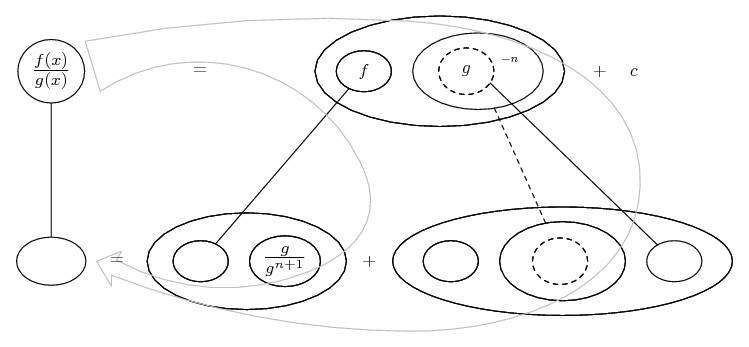Note: the function 1/g would be written g/g2 in the bottom row, just so we have a common denominator ready for simplifying the row... very like the standard quotient-rule formula. But, note also that if g is a power of a function (e.g. if it's sin2x) then we'll put sinx in the dashed balloon and write the second balloon in the bottom row as sinx over sin3x. This is (in these cases) a bit neater than the formula, which produces sin4x in the denominator.

Examples of differentiating a quotient (2x4 - 3x) / (4x - 1) 1/6 e2 sin x - 3x / (3 + 2 cos x) (1 - x2)3 / (6 + 2x)3 (2x - 3)2 / (x3 - 7)3 x (x2)/(x + 2) (1 - x2)/(x2 + 1)2 (x + 5)/(2x - 4) -4x/(x2 - 1)2 (1 + x2)/(1 + 2x2) 5 cosx/(4 - secx) x/(x + 2)2 x2/e-2x tan t (exponential form) ln|x| / √x √(7x - 2) / √(5x + 3) (4x + 3)7 / (5x - 2)7 3x/(x2 + 4) (4 - x2) / [ (x2 + 4) ]2 (1-s2 ) / (1 + s2 )2

##### Differentiate an inverse trig functionSimilarly, to justify the general formula for differentiating an inverse: d/dx f  -1(x) = 1 / [f '(f  -1(x))]...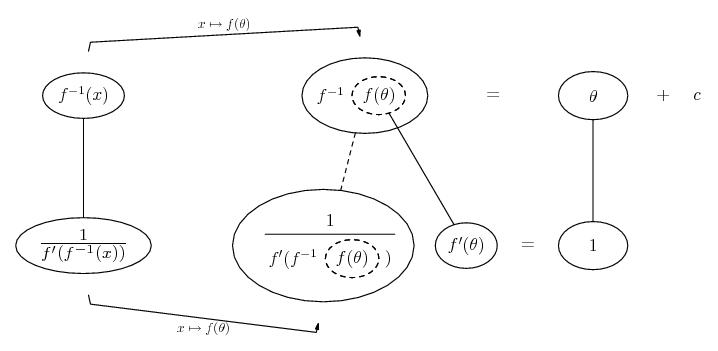##### Separation of variables

Get your equation in the form f(y) * dy/dx = f(x). (Not a complete separation). Fit the equation along the bottom row...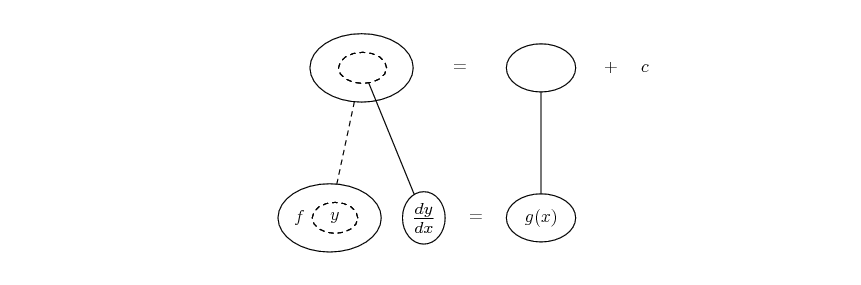You can then extend the diagram on either side if necessary (chain-rule shapes shown for example).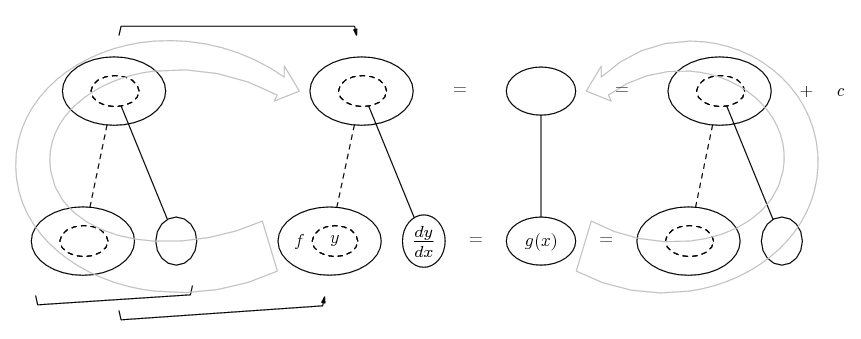##### Integrating factor

If you can get your equation in the form      dy/dx + y f(x) = 0      or      dy/dx + y f(x) = g(x),

then fill just these places in the bottom row...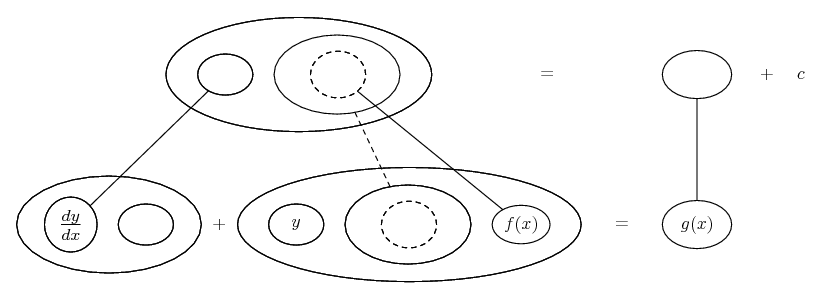... and the others will follow...Note: in place of y you can accomodate a function f(y)... as long as, in the place of dy/dx, you have d (f(y)) / dx.

Examples of choosing an integrating factor (x3 + y) dx - x dy = 0 birth and death rates ex dy/dx = 1 - 2exy dy/dx = 2x (1 + x2 - y) dy/dx = y/x + y2/x2 x dy/dx - 4y = 0 dθ/dt + θ/10 = 5 - 5t/2 x2 y' + xy = x ex dy/dx - y / (x + 1) = x - 1

##### Exact equations

If you can get the left-side of your equation to fit the lower level of the product rule without need of an integrating factor (see above), the equation is exact. Most examples of 'exact equations', however, don't necessarily fit the product rule as such, but do fit the multi-variable chain rule...... of which the product rule is a special case. To be able to find F you need an equation that fits the bottom row and also passes the 'exactness test'. That is, you need to check that the partial derivative of M with respect to y equals that of N with respect to x. That settled, you know you can integrate as follows...

##### Reduction of Order##### Fundamental theorem of calculus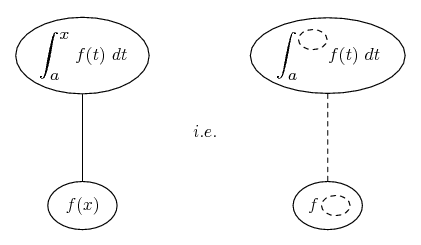##### Double and Triple Integrals##### Differentiation Under the Integral Sign